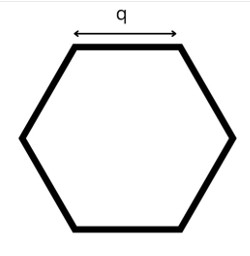# Swift Program to Calculate Area of Hexagon

This tutorial will discuss how to write swift program to calculate area of hexagon.

A hexagon is a two-dimensional shape with 6 sides of same or different length. It contains six vertices and six interior angles. Hexagon is classified into four types according to their length and internal angles −

• Regular Hexagon
• Irregular Hexagon
• Convex Hexagon
• Concave Hexagon

In this article we will find the area of Regular hexagon. Regular hexagon are those hexagons in which the all the sides are of equal length. The sum of all six interior angles is 720 degrees and the sum of all six exterior angles is 360 degrees.

The total amount of space enclosed inside the six sides of the regular hexagon is known as the area of the hexagon.## Formula:

Following is the formula of the area of the hexagon −

Area = (3 √3(q)2 ) / 2


Below is a demonstration of the same −

Input

Suppose our given input is −

side = 10


Output

The desired output would be −

Area of hexagon = 259.8076211353316


## Algorithm

Following is the algorithm −

Step 1 - Create a function with return value.

Step 2 - Find the area of the hexagon using the following formula −

return (3*sqrt(3)*q*q)/2


Step 3 - Calling the function and pass the side in the circle as a parameter.

Step 4 - Print the output.

## Example

The following program shows how to calculate the area of hexagon.

import Foundation
import Glibc

// Creating a function to find the area of hexagon
func hexagonArea(q:Double) -> Double{
return (3*sqrt(3)*q*q)/2
}
var num = 15.0
print("Length of the side is", num)
print("Area of the hexagon:", hexagonArea(q:num))


## Output

Length of the side is 15.0
Area of the hexagon: 584.5671475544962


Here, in the above program we create a function which return the area of the hexagon using the following formula −

return (3*sqrt(3)*q*q)/2


Here, we use sqrt() function to find the square root of 3.

Updated on: 30-Nov-2022

121 Views